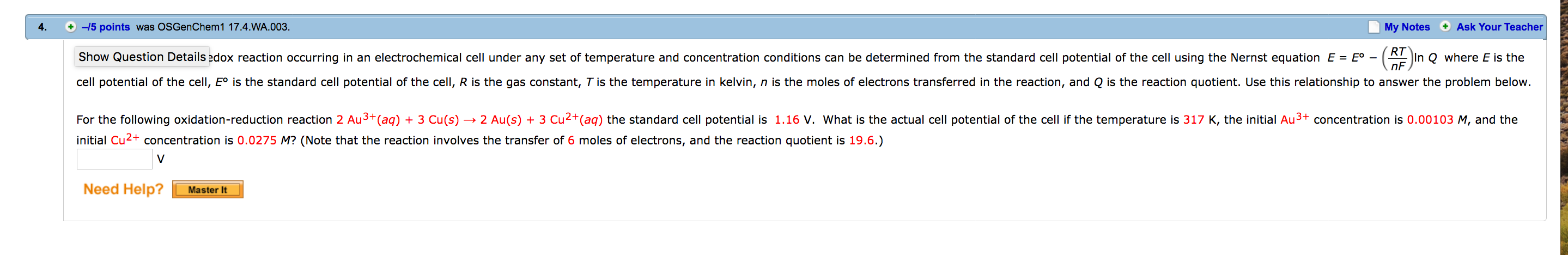# A redox reaction occurring in an electrochemical cell under any set of temperature and concentration conditions can be determined from the standard cell potential of the cell using the Nernst equation E = Eo - (RT/nF)lnQ where E is the cell potential of the cell, Eo is the standard cell potential of the cell, R is the gas constant, T is the temperature in kelvin, n is the moles of electrons transferred in the reaction, and Q is the reaction quotient. Use this relationship to answer the problem below.